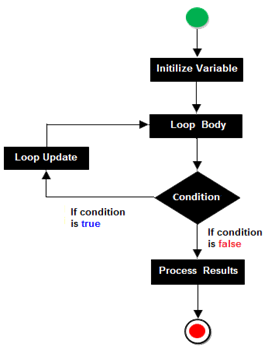#### Do While loop

The do while loop is an exit controlled loop, since the body of loop is executed first and then the test condition is evaluated. If it evaluates to false, then the loop is terminated, otherwise it is repeated. This means do while loop always executes at least once, even if the condition is evaluated to false.

Syntax:`do{	statement 1;	statement 2;	…………….	statement N;}while(condition);`

#### Example Programs

Program 

Write a program to input a number and check whether it is a happy number or not. If you iterate the process of summing the squares of the decimal digits of a number and if this process terminates in 1, then the original number is called a happy number. For example 7=> (72)=49=> (42+92)=97=>(92 +72)=130 =>(12 +32+02)=10 =>(12+02)= 1.

Program 

Write a program in Java to print the sum of negative numbers, sum of positive odd numbers and sum of positive even numbers from a list of numbers entered by the user. The list terminates when the user enters a Zero.